## 人工神经网络之乳腺癌识别_Sim1480的博客-程序员宅基地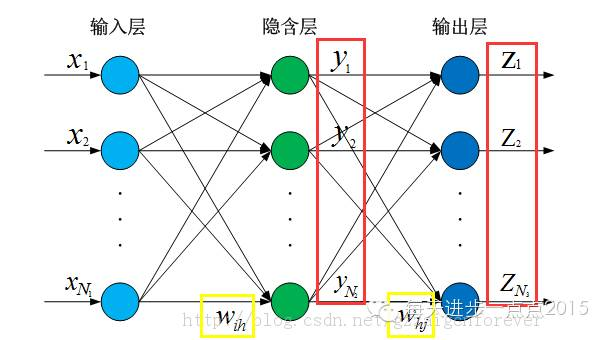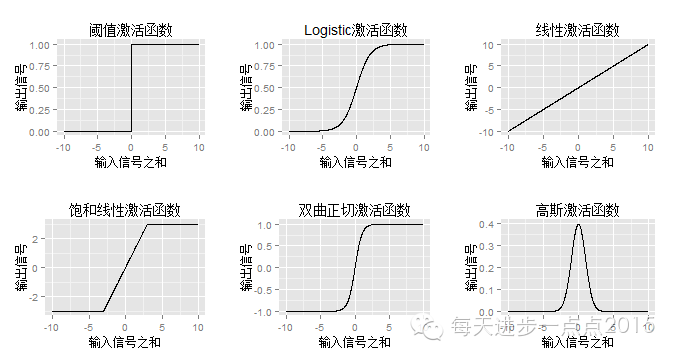standard1 <- function(x){

(x-min(x))/(max(x)-min(x))

}

standard2 <- function(x){

(x-mean(x))/sd(x)

}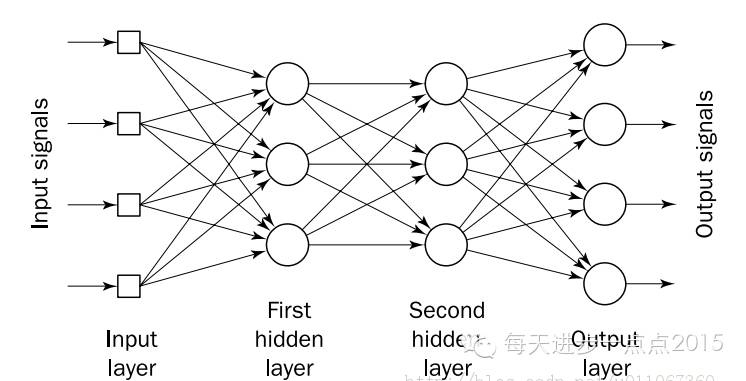1）适用于分类和数值预测问题

2）对数据几乎不作任何假设条件

1）计算量大、训练缓慢，尤其是网络拓扑结构相当复杂时

2）容易发生过拟合

3）输出结果很难解释

nnet包中的函数nnet()语法：

nnet(formula, data, weights, ...,

subset, na.action, contrasts = NULL)

nnet(x, y, weights, size, Wts, mask,

linout = FALSE, entropy = FALSE, softmax = FALSE,

censored = FALSE, skip = FALSE, rang = 0.7, decay = 0,

maxit = 100, Hess = FALSE, trace = TRUE, MaxNWts = 1000,

abstol = 1.0e-4, reltol = 1.0e-8, ...)

formula：模型的公式表达形式，类似于y~x1+x2+x3

data：指定要分析的数据对象

weights：代表各类样本在模型中所占比重，默认将各类样本按原始比重建立模型

subset：可提取目标数据集的子集作为模型的训练样本

na.action：处理缺失值的方法，默认忽略缺失值

x：为输入的自变量矩阵或数据框

y：为输入的因变量，但必须经过class.ind()函数的预处理

size：指定隐藏层节点个数，通常为输入变量个数的1.2至1.5倍

Wts：设置初始的权重，默认情况将随机产生权重值

linout：指定线性输出还是Logistic输出，默认为Logistic输出

rang：设置初始权重值的范围[-rang,rang]

decay：指模型建立过程中，模型权重值的衰减精度，默认为0

maxit：指定模型的最大迭代次数

RSNNS包中的mlp()函数--多层前馈网络

mlp(x, y, size = c(5), maxit = 100,

initFunc = "Randomize_Weights", initFuncParams = c(-0.3, 0.3),

learnFunc = "Std_Backpropagation", learnFuncParams = c(0.2, 0),

updateFunc = "Topological_Order", updateFuncParams = c(0),

hiddenActFunc = "Act_Logistic", shufflePatterns = TRUE, linOut = FALSE,

inputsTest = NULL, targetsTest = NULL, pruneFunc = NULL,

pruneFuncParams = NULL, ...)

x：为输入的自变量矩阵或数据框

y：为输入的因变量

size：指定每个隐藏层的节点数，默认是单层5节点的拓扑结构

maxit：指定模型的最大迭代次数

initFunc：指定权重的初始函数

initFuncParams：权重的初始值默认在(-0.3, 0.3)之间

learnFunc：指定计算神经网络的算法类型，默认为标准后向传播算法

learnFuncParams：指定学习算法参数的初始值，即学习速率和最大输出误差

updateFunc：指定替换的算法类型

hiddenActFunc：指定隐藏层的算法类型

linOut：指定输出层的激活函数，可以是线性或Logistic

neuralnet包中的neuralnet()函数语法

neuralnet(formula, data, hidden = c(1), threshold = 0.01,

stepmax = 1e+05, rep = 1, startweights = NULL,

learningrate.limit = NULL,

learningrate.factor = list(minus = 0.5, plus = 1.2),

learningrate=NULL, lifesign = "none",

lifesign.step = 1000, algorithm = "rprop+",

err.fct = "sse", act.fct = "logistic",

linear.output = TRUE, exclude = NULL,

constant.weights = NULL, likelihood = FALSE)

formula：模型的公式表达形式，类似于y~x1+x2+x3，不允许y~.的格式

data：指定要分析的数据对象

hidden：指定每个隐藏层的节点数，默认是单层1节点的拓扑结构

threshold：指定误差函数的偏差阈值，默认为0.01

stepmax：指定模型的最大迭代次数

rep：指定神经网络训练的次数

startweights：设置初始的权重，默认情况将随机产生权重值

learningrate.limit：指定学习速率的最小最大值，该参数仅对RPROP和 GRPROP方法起效

learningrate：可为后向传播算法指定学习速率

algorithm：指定计算神经网络的算法类型

#读取数据

str(cancer)

#数据标准化

cancer_stand <- sapply(cancer[,-c(1,2)], standard1)

#数据合并

cancer_stand <- as.data.frame(cbind(diagnosis = cancer\$diagnosis, cancer_stand))

#将目标变量转换为因子

cancer_stand\$diagnosis <- factor(cancer_stand\$diagnosis, levels = c(1,2), labels = c('B','M'))

#构建训练样本集和测试样本集

set.seed(1234)

index <- sample(c(1,2), nrow(cancer_stand), replace = TRUE, prob = c(0.8,0.2))

train <- cancer_stand[index == 1,]

test <- cancer_stand[index == 2,]

#使用nnet包中的nnet()函数建模

library(nnet)

#通过循环，确定最佳的节点数

err1 <- 0

err2 <- 0

for (i in 1:45){

set.seed(1234)

model <- nnet(diagnosis ~ ., data = train, maxit = 300, size = i, trace = FALSE)

err1[i] <- sum(predict(model, train, type = 'class') != train\$diagnosis)/nrow(train)

err2[i] <- sum(predict(model, test, type = 'class') != test\$diagnosis)/nrow(test)

}

plot(err1, type = 'b', col = 'black', lty = 2, lwd = 2, ylab = '误差', xlab = '节点数', ylim = c(0,0.05), pch = 10)

lines(err2, type = 'b', col = 'blue', lty = 2, lwd = 2, pch = 23)

legend(locator(1), legend = c('训练集误差率','测试集误差率'), col = c('black','blue'), lty = c(2,2), lwd = c(2,2), bty = 'n',

pch = c(10,23))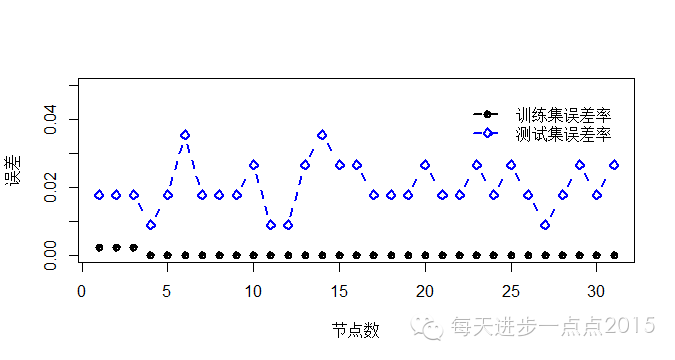#通过循环，确定最大迭代次数

err1 <- numeric()

err2 <- numeric()

for (i in 1:500){

set.seed(1234)

model <- nnet(diagnosis ~ ., data = train, maxit = i, size = 4, trace = FALSE)

err1[i] <- sum(predict(model, train, type = 'class') != train\$diagnosis)/nrow(train)

err2[i] <- sum(predict(model, test, type = 'class') != test\$diagnosis)/nrow(test)

}

plot(err1, type = 'l', col = 'black', lty = 1, ylab = '误差', xlab = '节点数')

lines(err2, type = 'l', col = 'blue', lty = 4)

legend(locator(1), legend = c('训练集误差率','测试集误差率'), col = c('black','blue'), lty = c(1,4), bty = 'n')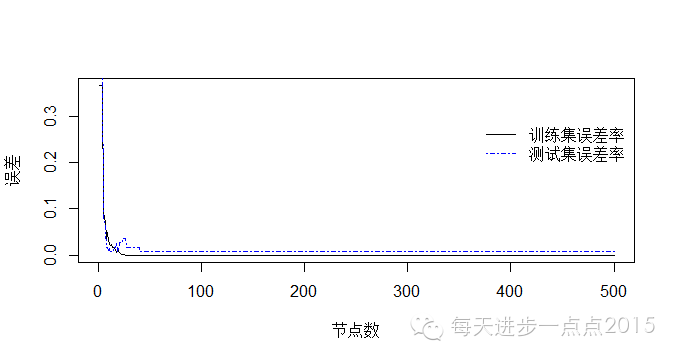#建立最终的神经网络模型

set.seed(1234)

model_nnet <- nnet(diagnosis ~ ., data = train, maxit = 50, size = 4, trace = FALSE)

pred_nnet <- predict(model_nnet, test, type = 'class')

#预测精度

Freq_nnet <- table(test\$diagnosis, pred_nnet)

Freq_nnet

accuracy_nnet <- sum(diag(Freq_nnet))/sum(Freq_nnet)

accuracy_nnet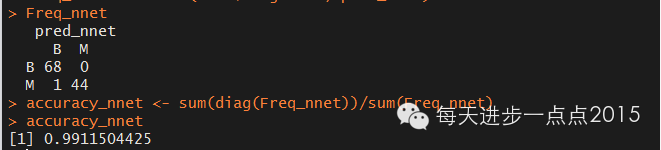#使用RSNNS包中的mlp()函数建模

library(RSNNS)

#将数据顺序打乱

data_cancer = cancer[sample(1:nrow(cancer),length(1:nrow(cancer))),2:ncol(cancer)]

#定义网络输入

cancerValues= data_cancer[,-1]

#定义网络输出，并将数据进行格式转换

cancerTargets = decodeClassLabels(data_cancer[,1])

#从中划分出训练样本和检验样本

set.seed(1234)

model_cancer = splitForTrainingAndTest(cancerValues, cancerTargets, ratio=0.20)

#数据标准化

model_cancer = normTrainingAndTestSet(model_cancer, type = '0_1')

#利用mlp命令执行前馈反向传播神经网络算法

model_mlp = mlp(model_cancer\$inputsTrain, model_cancer\$targetsTrain, size=4, maxit=100, inputsTest=model_cancer\$inputsTest, targetsTest=model_cancer\$targetsTest)

#利用上面建立的模型进行预测

pred_mlp = predict(model_mlp, model_cancer\$inputsTest)

#生成混淆矩阵，观察预测精度

Freq_mlp <- confusionMatrix(model_cancer\$targetsTest,pred_mlp)

Freq_mlp

accuracy_mlp <- sum(diag(Freq_mlp))/sum(Freq_mlp)

accuracy_mlp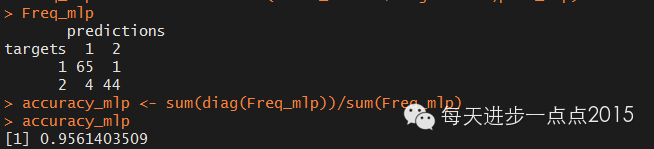sapply()

nnet包

nnet()

predict()

table()

RSNNS包

decodeClassLabels()

splitForTrainingAndTest()

normTrainingAndTestSet()

mlp()

confusionMatrix()

neuralnet包

neuralnet()

compute()

http://ju.outofmemory.cn/entry/84442

http://www.r-bloggers.com/fitting-a-neural-network-in-r-neuralnet-package/

### JAVA 逆序_逆序java-程序员宅基地

54321 =》12345有这样的一个规则:JAVA:java的/取的是整数部分1%10=120/10%10=230/10/10%10=340/10/10/10%10=450/10/10/10%10=5public class arrx { public static void main(String[] args){ _逆序java

### 动态执行程序-程序员宅基地

1,语法检查EDITOR_SYNTAX_CHECK2,程序生成 GENERATE SUBROUTINE POOL itab_prog NAME prog MESSAGE msg.

### 文本分类-必读论文合集推荐-AMiner-程序员宅基地

AMiner平台由清华大学计算机系研发，拥有我国完全自主知识产权。平台包含了超过2.3亿学术论文/专利和1.36亿学者的科技图谱，提供学者评价、专家发现、智能指派、学术地图等科技情报专业化服务。系统2006年上线，吸引了全球220个国家/地区1000多万独立IP访问，数据下载量230万次，年度访问量超过1100万，成为学术搜索和社会网络挖掘研究的重要数据和实验平台。AMiner平台：https://www.aminer.cn文本分类用电脑对文本集(或其他实体或物件)按照一定的分类体系或标准进行自动分.

### 使用for循环输出一个倒三角形。_for循环输出倒三角-程序员宅基地

// 使用for循环输出一个倒三角形。for (int i = 0;i < 10;i++){for (int j = 10;j >= i;j–){System.out.print(" “);}for (int c = 1;c <= i;c++){System.out.print(”*");}System.out.print("\n");}输出结果：_for循环输出倒三角